# DLTK's Educational Activities for KidsOther Shapes Practice Crafts

Cut and paste craft templates to help young children learn their shapes.  Suitable for toddlers and preschoolers, but also loads of fun for kindergarten kids, early grade school children and moms *wink*.  This page includes all of the shapes crafts except for the Shapes Buddies.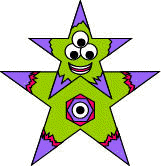### Alien Shapes Practice Paper Craft

Age 2+

(practice stars)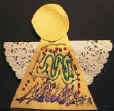### Angel Shapes Practice Paper Craft

Age 2+

(practice circles and triangles)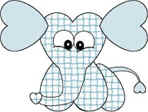### Animal Hearts Paper Crafts

Age 2+

(practice hearts)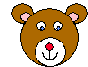### Bear Circle Practice Paper Craft

Age 2+

(practice circles)### Beaver Circle Practice Paper Craft

Age 2+

(practice circles and squares)### Black Panther Shapes Practice Paper Craft

Age 2+

(practice circles)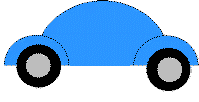### Car Shapes Practice Paper Craft

Age 2+

(practice circles and triangles)### Cat Shapes Practice Paper Craft

Age 2+

(practice circles and triangles)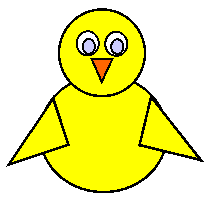### Chick Shapes Practice Paper Craft

Age 2+

(practice circles and triangles)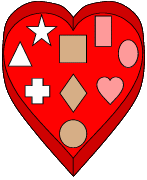### Chocolate Box Shapes Practice Paper Craft

Age 2+### Dog Circles Practice Paper Craft

Age 2+

(practice circles)### Elephant Shapes Practice Paper Craft

Age 2+

(practice circles, rectangles and triangles)### Fish Triangle Practice Paper Craft

Age 2+

(practice triangles)### Fox Shapes Practice Paper Craft

Age 2+

(practice stars)### Frog Shapes Practice Paper Craft

Age 2+

(practice circles)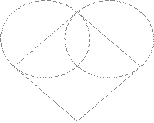### Heart with Circles and a Square

Age 5+### Horse Shapes Practice Paper Craft

Age 2+

(practice ovals, rectangles and triangles)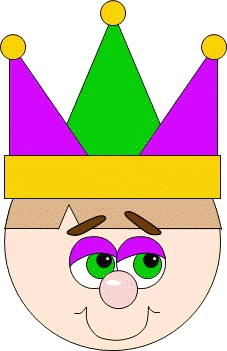### Jester Circles and Triangles Practice Paper Craft

Age 2+

(practice circles and triangles)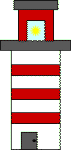### Lighthouse Rectangles Practice Paper Craft

Age 2+

(practice rectangles)### Lamb Circles Practice Paper Craft

Age 2+

(practice circles)### Lion Circles Practice Paper Craft

Age 2+

(practice circles)### Owl Shapes Practice Paper Craft

Age 2+

(practice circles, ovals and triangles)### Peacock Paper Craft

Ages 2+

(practice circles, ovals and triangles)### Pig Paper Craft

Ages 2+

(practice circles, ovals and triangles)### Pink Panther Circles Practice Paper Craft

Age 2+

(practice circles)### Polar Bear Circles Practice Paper Craft

Age 2+

(practice circles)### Pumpkin Shapes Practice Paper Craft

Age 2+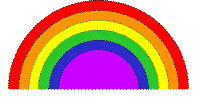### Rainbow Paper Craft

Age 2+

(practice circles)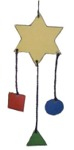### Shapes Mobile

Age 2+

(practice circles, squares, triangles, and stars)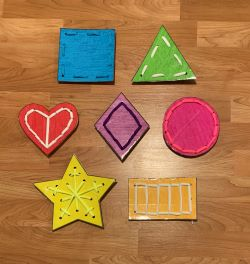### Shapes Sewing

Age 5+

(practice shapes and colors for circles, squares, triangles, rectangles, diamonds, hearts, and stars)### Schoolhouse Shapes Paper Craft

Age 2+

(practice rectangles, triangles, and squares)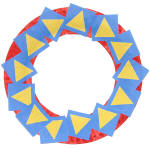### Shapes Paper Plate Wreath

Age 3+

(practice circles, triangles, and squares)### Snakes Shapes Practice Crafts

Age 2+

(practice circles, diamonds, hearts, ovals, rectangles, squares and triangles)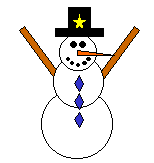### Snowman Shapes Practice Paper Craft

Age 2+

(practice circles, rectangles, diamonds, triangles, squares and stars)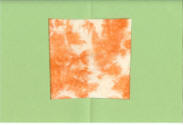### Stained Glass Shapes Crafts

Age 4+

(practice circles, rectangles, diamonds, triangles, and squares)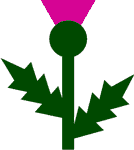### Thistle Shapes Practice Paper Craft

Age 2+

(practice circles, rectangles and triangles)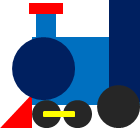### Train Shapes Practice Paper Craft

Age 2+

(practice circles, rectangles and triangles)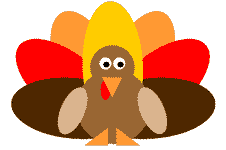### Turkey Shapes Practice Paper Craft

Age 2+

(practice circles, ovals and triangles)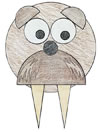### Walrus Shapes Practice Paper Craft

Age 2+

(practice circles, half circles and triangles)